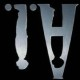# 从8和9说起$\displaystyle{b\ln(y) < \ln(y^b+1) < b\ln(y) + y^{-b}}$$\displaystyle{|a \ln(x) - b \ln(y)| < y^{-b}}$$C(x,y,a,b) < |a \ln(x) - b \ln(y)|$$\displaystyle{e^{e^{e^{e^{730}}}}}.$# 从整数到多项式$\displaystyle{P = \frac{1}{27} t^{15} + \frac{1}{3} t^{12} + \frac{4}{3} t^9 + \frac{8}{3} t^6 + \frac{5}{2} t^3 + \frac{1}{2}}$$\displaystyle{Q = \frac{1}{9} t^{10} + \frac{2}{3} t^7 + \frac{5}{3} t^4 + \frac{4}{3} t}$$\displaystyle{R = \frac{1}{36} t^6 + \frac{7}{54} t^3 + 4}$$\displaystyle{P = x^{15} - 3x^{14} + 51x^{13} - 67x^{12} + 969x^{11} + 33x^{10} + 10963x^9 + 9729x^8 + 96507x^7}$$\displaystyle{\quad + 108631x^6 + 580785x^5 + 700503x^4 + 2102099x^3 + 1877667x^2 + 3904161x + 1164691}$$\displaystyle{Q = x^{10} - 2x^9 + 33x^8 - 12x^7 + 378x^6 + 336x^5 + 2862x^4 + 2652x^3 + 14397x^2 + 9922x + 18553}$$\displaystyle{R = - 2^6 3^{15}(5x^6 - 6x^5 + 111x^4 + 64x^3 + 795x^2 + 1254x + 5477)}$

# 球面覆盖$\displaystyle{f(x) = -\frac{(x-1)^3 (x-9)}{64x}}$$\displaystyle{f(x) - 1 = -\frac{(x^2-6x-3)^2}{64x}}$$\displaystyle{z \mapsto \frac{az+b}{cz+d}},$

# 小朋友的涂鸦$\displaystyle{f(x) = -\frac{(x-1)^3 (x-9)}{64x}}$0处的分支点 黑色顶点
1处的分支点 白色顶点
∞处的分支点
0处和1处分支点的重数 顶点的度数
∞处分支点的重数 面的度数的一半# 地图的魔术

0处的分支点 黑色顶点
1处的分支点 白色顶点
∞处的分支点
0处和1处分支点的重数 顶点的度数
∞处分支点的重数 面的度数的一半$\displaystyle{v-e+f=2}.$k=5的四组解，蒙A. Zvonkine惠允

# 一个规划的大纲“由于目前在大学里教学和研究方面的结合于我而言愈发虚无飘渺，我决定申请加入CNRS，为的是能够将我的精力奉献于发展某些工作和视点，因为现在来看，明显以后找不到会代替我发展它们的学生（似乎连同行的数学家也没有）。”

（Comme la conjoncture actuelle rend de plus en plus illusoire pour moi les perspectives d’un enseignement de recherche à l’Université, je me suis résolu à demander mon admission au CNRS, pour pouvoir consacrer mon énergie à développer des travaux et perspectives dont il devient clair qu’il ne se trouvera aucun élève (ni même, semble-t-il, aucun congénère mathématicien) pour les développer à ma place.）Teichmüller层级（tour de Teichmüller）、绝对伽罗华群$\mathrm{Gal}(\overline{\mathbb{Q}} / \mathbb{Q})$的作用、有限域上的正则多面体、驯顺拓扑（topologie modérée）……他笔下倾泻出近年他关心的数学领域和数学对象，这一写就是48页，还没算上注记。

“这样的假设当时似乎很离谱，我甚至不敢向这方面的行家询问这个问题。我问过德利涅，他也觉得确实很离谱，但手头上没有反例。不到一年之后，在赫辛斯基的国际数学家大会上，苏联数学家别雷就宣布了这个结果，他的证明简洁得不合常理，在德利涅的信里只占了两页——也许从来没有过如此深刻而奇妙的结果能用那么少的行数来证明！”

（Une telle supposition avait l’air à tel point dingue que j’étais presque gêne de la soumettre aux competences en la matière. Deligne consulté trouvait la supposition dingue en effet, mais sans
avoir un contre-exemple dans ses manches. Moins d’un an après, au Congrès International de Helsinki, le mathematicien sovietique Bielyi annonce justement ce résultat, avec une demonstration d’une simplicite deconcertante tenant en deux petites pages d’une lettre de Deligne – jamais sans doute un résultat profond et déroutant ne fut demontre en si peu de lignes!）# 失之东隅# 后记

Merci beaucoup Sacha ! Bonne retraite !Alexandre Zvonkine，图片来自他的学术主页https://www.labri.fr/perso/zvonkin/

# 参考文献

Alexandre Zvonkine. Weighted trees, Journées Combinatoires de Bordeaux, 2016

Sergey Lando and Alexander Zvonkin. Graphs on surfaces and their applications, volume 141 of Encyclopaedia of Mathematical Sciences. Springer-Verlag, 2004.

Alexandre Grothendieck. Esquisse d’un programme, 1984

Allyn Jackson. Comme Appelé du Néant —— As If Summoned from the Void: The Life of Alexandre Grothendieck, Notices of the AMS, Volume 51, Number 9 and 10, 2004. Part 1, Part 2

Wenjie Fang. Aspects énumératifs et bijectifs des cartes combinatoires : généralisation, unification et application, PhD Thesis, 2016.

## “小朋友的涂鸦”的一个响应

1.smtptty说道：

波澜壮阔

2. Pingback引用通告： 解构与复原：望月新一与他的证明 | fwjmath的相空间